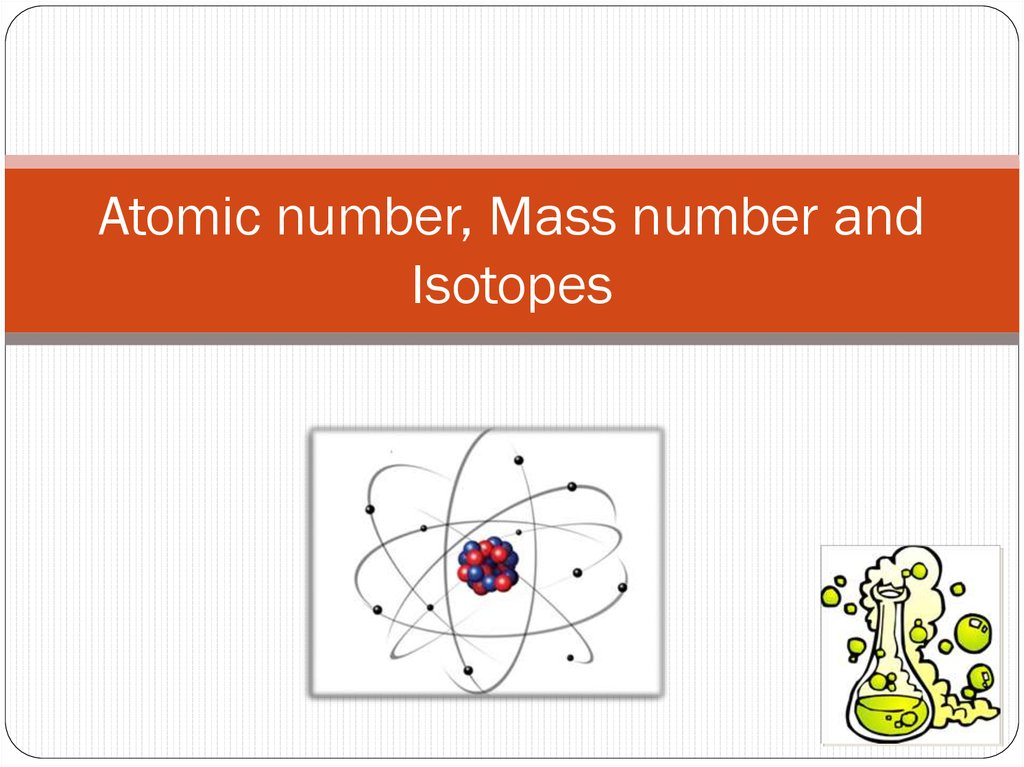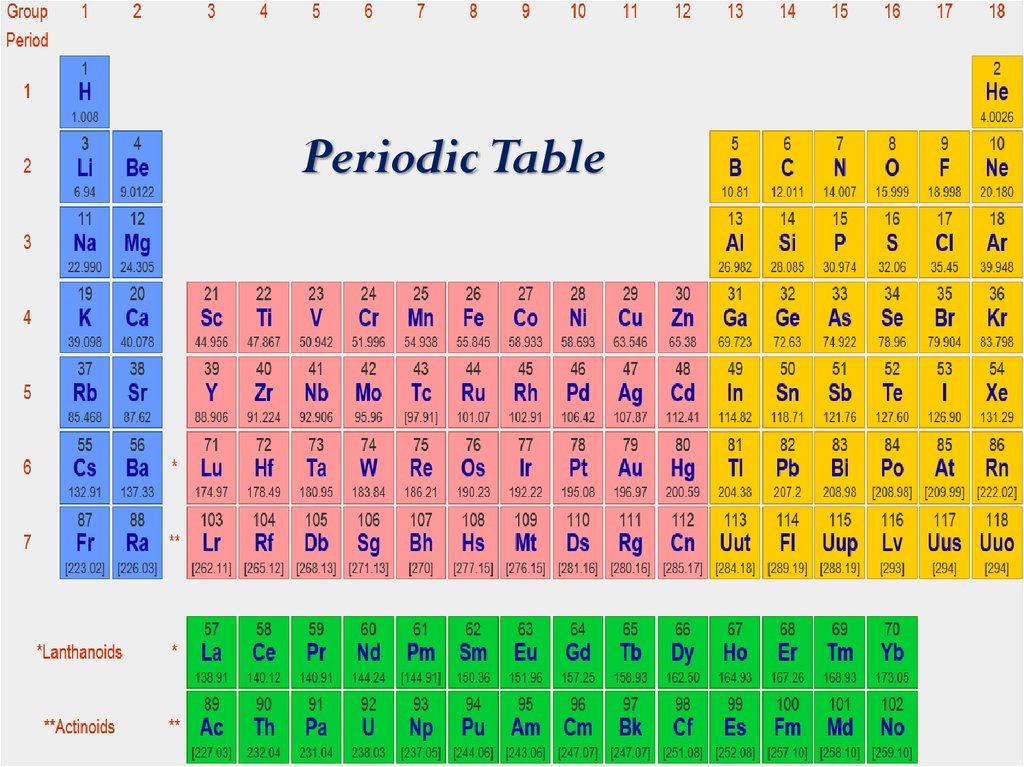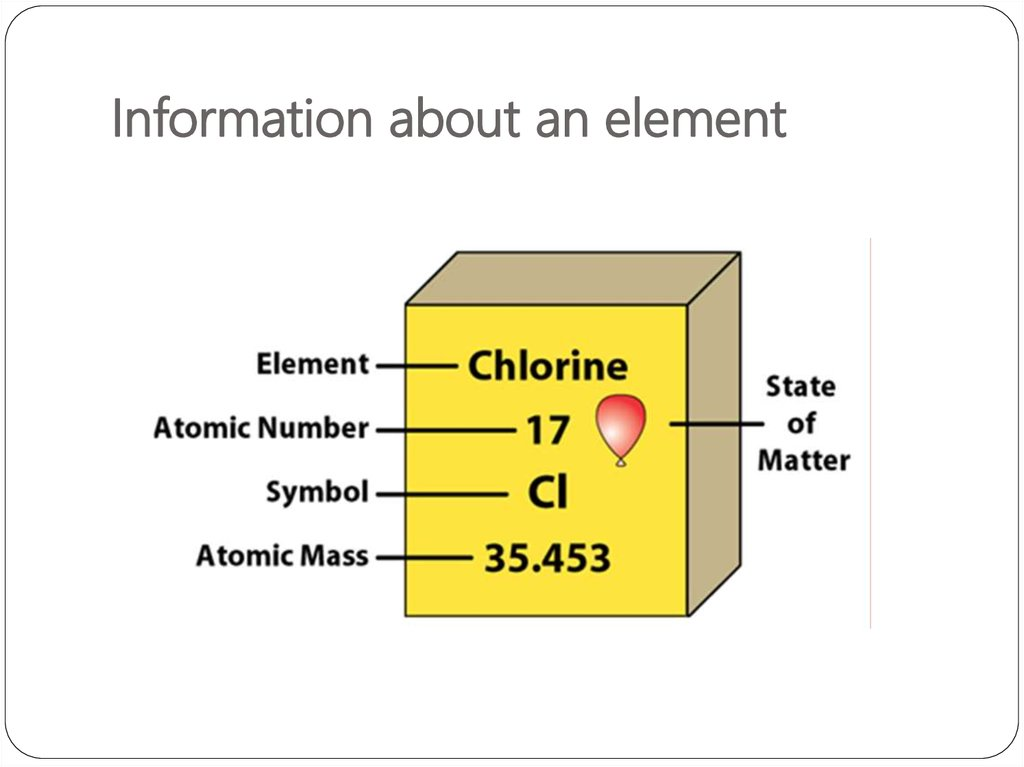# Atomic number, Mass number and Isotopes

Periodic Table

## 4.

Let’s take Li atom for an example:
It has 3 protons, 3 neutrons and 3 electrons.
What are its atomic number and mass number?

## 5. Atomic number and Mass Number

The number of protons in a given atom is called the
atomic number, or the proton number, Z.
The number of electrons in the atom is also equal to
Z
because the atom is electrically neutral.
The total number of protons and neutrons in an atom
is called the mass number, A.
The number of neutrons, the neutron number, is A - Z.
An electron carries an atomic unit of negative charge,
a proton carries an atomic unit of positive charge, and
a neutron is electrically neutral.

## 6. Atomic number and Mass Number

Example:
Atomic Number = 17
Mass Number = 36
Neutron number = 19

## 7. Number of electrons

An atom is neutral.
The net charge is zero.
Therefore, Number of protons = Number of electrons
Atomic number = Number of electrons
For chlorine (Cl),
Atomic number = Number of electrons = 17

## 13. Isotopes同位素

They are atoms of the same element that have
different numbers of neutrons.
Hydrogen has 3 isotopes.
Note how the number of
protons never change!!!

## 14. Isotopes

Hydrogen has 3 isotopes.
Have more neutrons,
increases the mass.

## 15. Isotopes

Hydrogen has 3 isotopes.
Mass number = 2

## 16.

Do You Understand Isotopes?
How many protons, neutrons, and electrons are in
14
C ?
6
6 protons, 8 (14 - 6) neutrons, 6 electrons
How many protons, neutrons, and electrons are in
6 protons, 5 (11 - 6) neutrons, 6 electrons
11
C
6 ?

Periodic Table

## 23. Cation and Anion

An
ion is an atom, or group of atoms, that has a net
positive or negative charge.
cation – ion with a positive charge
If a neutral atom loses one or more electrons it becomes a
cation.
Na
11 protons
11 electrons
Na+
11 protons
10 electrons
anion – ion with a negative charge
If a neutral atom gains one or more electrons it becomes
an anion.
Cl
17 protons
17 electrons
Cl-
17 protons
18 electrons

## 24. Cation and Anion

A
monatomic ion contains only one atom
eg. Na+, Cl-, Ca2+, O2-, Al3+, N3 A polyatomic ion contains more than one atom
eg. OH-, CN-, NH4+, NO3-

## 25.

Do You Understand Ions?
3+
27
How many protons and electrons are in
Al ?
13
13 protons, 10 (13 – 3) electrons
How many protons and electrons are in 78 Se2-?
34
34 protons, 36 (34 + 2) electrons

***

## 30. Why does the atomic mass on the periodic table have so many decimal places?

Different concept from Mass number!!!

## 31. Atomic mass

1
H
1.00797
• This atomic mass is the one number that best
represents the mass of all three versions of hydrogen.
• No atom of hydrogen anywhere in the universe actually
has this mass.
• This number is a “weighted” average of the masses of
every isotope of hydrogen. (Relative atomic mass)

## 32. Calculating a “weighted” average

1. First, you have to know the mass of each isotope.
2. Second, you have to know the percent abundance*
of each isotope.
* Percent abundance is how often that particular
isotope occurs in a sample of the element.

## 33. Calculating a “weighted” average

A sample of
magnesium collected
anywhere in the
universe will contain
three isotopes of
magnesium.
Sample of Magnesium (Mg)
78.90% of this magnesium will be the isotope named “magnesium 24”
10.00% of this magnesium will be the isotope named “magnesium 25”
11.10% of this magnesium will be the isotope named “magnesium 26”

Protons
Neutrons
Mass (amu)
%
abundance
Isotope 1
(magnesium
-24)
12
12
23.985045
78.90%
Isotope 2
(magnesium
-25)
12
13
24.985839
10.00%
Isotope 3
(magnesium
-26)
12
14
25.982595
11.10%

## 35. Calculating a “weighted” average

Atomic mass of magnesium
= 23.985045 x 78.90%
+ 24.985839 x 10.00%
+ 25.982595 x 11.10%
= 24.30685245 amu
* One atomic mass unit (1 amu) is exactly 1/12 the
mass of a carbon-12 atom.

## 37. Another example

natural chlorine.
• This atomic mass is the one number that best
represents the mass of all three versions of hydrogen.
37

## 38. Exercise

Copper has two isotopes 63Cu (62.9 amu) and 65Cu (64.9
amu). What is the % abundance of isotope 63Cu? (Hint:
Check periodic table for atomic mass)
1) 30%
2) 70%
3) 100%

Periodic Table

Periodic Table

## 42.

species of the same chemical element with different masses
whose nuclei are unstable and dissipate excess energy by
spontaneously emitting radiation in the form of alpha, beta,
and gamma rays.
Radioactive isotopes have many useful applications. In
medicine, for example, cobalt-60 is extensively employed as
a radiation source to arrest the development of cancer.
In industry, radioactive isotopes of various kinds are used for
measuring the thickness of metal or plastic sheets.
Other significant applications include the use of radioactive
isotopes as compact sources of electrical power—e.g.
plutonium-238 in cardiac pacemakers and spacecraft.